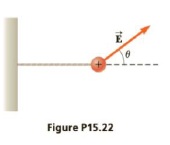Chapter 15, Problem 22P

Chapter
Section
Textbook Problem

A small sphere of charge q = +68 μC and mass m = 5.8 g is attached to a light string and placed in a uniform electric field E → . that makes ail angle θ = 37° with the horizontal. The opposite end of the string is attached to a wall and the sphere is in static equilibrium when the string is horizontal as in Figure P15.22. (a) Construct a free body diagram for the sphere. Find (b) the magnitude of the electric field and (c) the tension in the string.(a)

To determine
The free body diagram.

Explanation

The free body diagram is given by,

In the above diagram,

• T is the tension.
• m is the mass...

(b)

To determine
The magnitude of electric field.

(c)

To determine
The tension.

Still sussing out bartleby?

Check out a sample textbook solution.

See a sample solution

The Solution to Your Study Problems

Bartleby provides explanations to thousands of textbook problems written by our experts, many with advanced degrees!

Get Started